Author: Oscar Cronquist Article last updated on February 25, 2018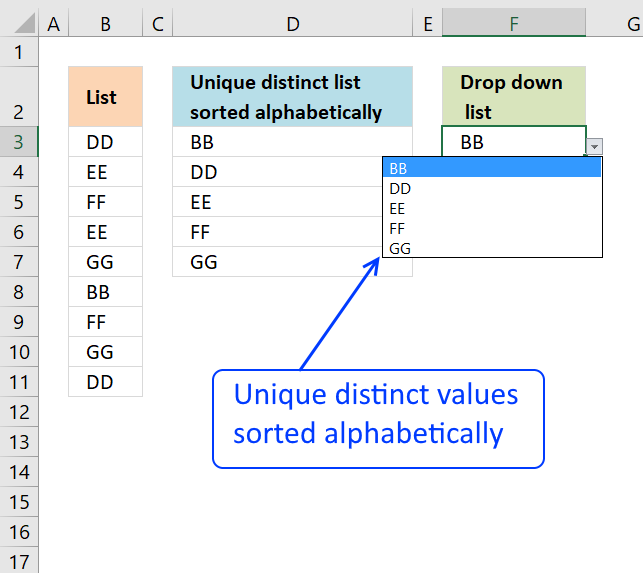Question:

How do I create a drop-down list with unique distinct alphabetically sorted values?

### Sort values using array formula

The helper column D filters unique distinct values sorted from A to Z from values in column B. The drop-down list in cell F3 is populated with the values in column D.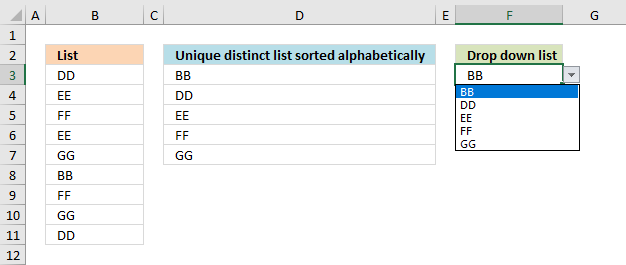Array formula in cell D3

=INDEX(\$B\$3:\$B\$11, MATCH(SMALL(IF(COUNTIF(\$D\$2:D2,\$B\$3:\$B\$11)=0,COUNTIF(\$B\$3:\$B\$11, "<"&\$B\$3:\$B\$11),""), 1), COUNTIF(\$B\$3:\$B\$11, "<"&\$B\$3:\$B\$11), 0))

#### Watch a video explaining how to populate Drop-Down list with a dynamic named range

Recommended article:

Sort a column alphabetically

Table of Contents Sort a column using array formula Two columns sorting by the second column Sort alphanumeric values I […]

Create dependent drop down lists containing unique distinct values

This article explains how to build dependent drop down lists. Here is a list of order numbers and products. We […]

How to create an array formula

1. Select cell D3
2. Type above array formula
3. Press and hold Ctrl + Shift
4. Press Enter once
5. Release all keys

Recommended article:

How to enter an array formula

Array formulas allows you to do advanced calculations not possible with regular formulas.

How to copy array formula

1. Select cell D3
2. Copy cell (Ctrl + c)
3. Select cell range D4:D7
4. Paste (Ctrl + v)

### Explaining array formula in cell D3

Step 1 - Filter unique distinct values

COUNTIF(\$D\$2:D2,\$B\$3:\$B\$11)=0

The COUNTIF function counts previous values above to make sure that no duplicates are displayed.

If no values in previous cells above are found the COUNTIF function returns a 0 (zero) for that position in the array.

COUNTIF(\$D\$2:D2,\$B\$3:\$B\$11) returns {0;0;0;0;0;0;0;0;0}

COUNTIF(\$D\$2:D2,\$B\$3:\$B\$11)=0 returns {TRUE;TRUE; TRUE;TRUE; TRUE;TRUE; TRUE;TRUE;TRUE}

The picture below shows the array in cell range C3:C11. The array tells the formula that no values have yet been displayed in cells above cell D3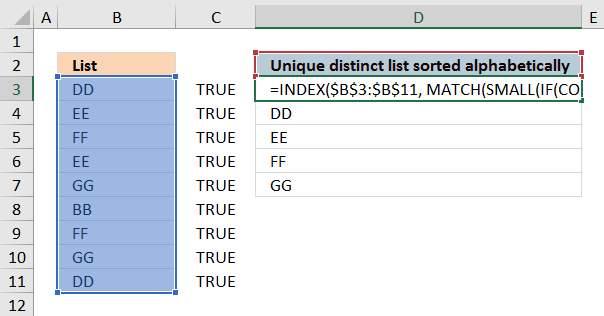Note that the first argument in the COUNTIF function (\$D\$2:D2) is a growing cell reference meaning when you copy the formula to cells below the cell reference expands automatically.

How to use the COUNTIF function

Counts the number of cells that meet a specific condition.

Step 2 - Create an array with sort rank numbers

The COUNTIF function comes once again to rescue, it allows you to rank each value in cell range B3:B11 based on its position in a sorted array.

COUNTIF(\$B\$3:\$B\$11, "<"&\$B\$3:\$B\$11)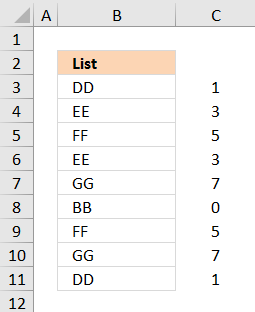Value "BB" is first in a sorted list indicated by the corresponding value 0 (zero) in column C. The second value is "DD" with rank number 1.

COUNTIF(\$B\$3:\$B\$11, "<"&\$B\$3:\$B\$11) returns {1;3;5;3;7;0;5;7;1}

Step 3 - Build an array with rank numbers based on which values that have not been shown yet

IF(COUNTIF(\$D\$2:D2,\$B\$3:\$B\$11)=0,COUNTIF(\$B\$3:\$B\$11, "<"&\$B\$3:\$B\$11),"")

becomes

IF({TRUE;TRUE; TRUE;TRUE; TRUE;TRUE; TRUE;TRUE;TRUE},{1;3;5;3;7;0;5;7;1},"")

and returns {1;3;5;3;7;0;5;7;1}

No values in the array have been filtered out, this makes sense since the array formula is in cell D3 and no values have been displayed yet.

How to use the IF function

Checks if a logical expression is met. Returns a specific value if TRUE and another specific value if FALSE.

Step 4 - Find the smallest value in the array

The SMALL function lets you filter the n-th smallest value from an array of values. It has a major advantage to the MIN function as it also ignores text and blank values.

SMALL(IF(COUNTIF(\$D\$2:D2,\$B\$3:\$B\$11)=0,COUNTIF(\$B\$3:\$B\$11, "<"&\$B\$3:\$B\$11),""), 1)

becomes

SMALL({1;3;5;3;7;0;5;7;1}, 1)

and returns 0 (zero).

How to use the SMALL function

The SMALL function lets you extract a number in a cell range based on how small it is compared to the other numbers in the group.

Step 4 - Find the position of a value in an array of values

To be able to fetch the right value to cell D3 we need to know it's position in column B. The MATCH function returns the position.

MATCH(SMALL(IF(COUNTIF(\$D\$2:D2,\$B\$3:\$B\$11)=0,COUNTIF(\$B\$3:\$B\$11, "<"&\$B\$3:\$B\$11),""), 1), COUNTIF(\$B\$3:\$B\$11, "<"&\$B\$3:\$B\$11), 0)

becomes

MATCH(0, COUNTIF(\$B\$3:\$B\$11, "<"&\$B\$3:\$B\$11), 0)

becomes

MATCH(0, {1;3;5;3;7;0;5;7;1}, 0)

and returns 6. Value 0 (zero) is in position 6 in this array {1;3;5;3;7;0;5;7;1}

How to use the MATCH function

Identify the position of a value in an array.

Step 5 - Fetch value

The INDEX function retrieves a value based on a coordinate, since it is only a column the INDEX function allows you to only use a row number as a coordinate.

INDEX(\$B\$3:\$B\$11, MATCH(SMALL(IF(COUNTIF(\$D\$2:D2,\$B\$3:\$B\$11)=0,COUNTIF(\$B\$3:\$B\$11, "<"&\$B\$3:\$B\$11),""), 1), COUNTIF(\$B\$3:\$B\$11, "<"&\$B\$3:\$B\$11), 0))

becomes

INDEX(\$B\$3:\$B\$11, 6)

becomes

INDEX({"DD";"EE";"FF";"EE";"GG";"BB";"FF";"GG";"DD"}, 6)

and returns value "BB" in cell D3.

How to use the INDEX function

Gets a value in a specific cell range based on a row and column number.

### How to create a drop-down list that expands with new values automatically

1. Click Data tab
2. Click Data Validation button
3. Click "Data validation..."
4. Select List in the "Allow:" window. See picture below.
5. Type =OFFSET(\$B\$2, 0, 0, COUNT(IF(\$B\$2:\$B\$1000="", "", 1)), 1) in the "Source:" window
6. Click OK!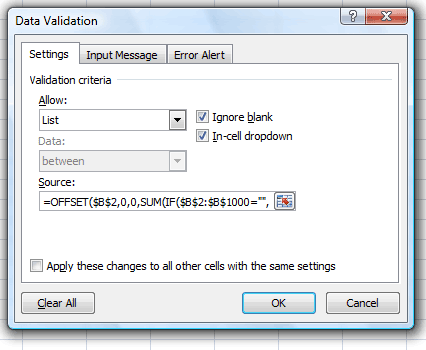Recommended articles:

Create dependent drop down lists containing unique distinct values

This article explains how to build dependent drop down lists. Here is a list of order numbers and products. We […]

### Final note

Convert the data in column B into an excel defined table to make it dynamic. Then you don't need to adjust the cell references in the array formula in column D every time you add or delete values in column B.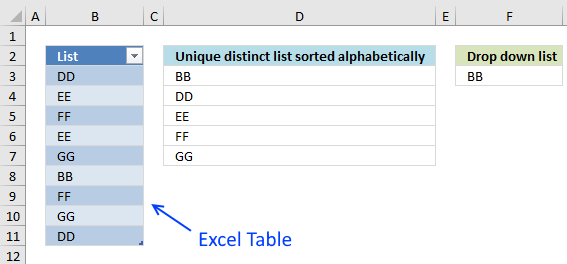Create a drop down list containing only unique.xlsx

Recommended articles:

Create dependent drop down lists containing unique distinct values in multiple rows

Sharmila asks: How can i use these list for multiple rows? I would like to use these lists for multiple […]

Invoice template with dependent drop down lists

This article demonstrates a basic invoice template I created. It lets you use dropdown lists to quickly select products on […]

Dependent drop down lists – Enable/Disable selection filter

Josh asks: I have this working right now with 6 drop downs/lists. I wanted to see if you possibly know […]

Dependent drop-down lists in multiple rows

This article demonstrates how to set up dependent drop-down lists in multiple cells. The drop-down lists are populated based on […]

IF(logical_test, [value_if_true],[value_if_false])
Checks whether a condition is met, and returns one value if TRUE, and another value if FALSE

INDEX(array,row_num,[column_num])
Returns a value or reference of the cell at the intersection of a particular row and column, in a given range

SMALL(array,k) returns the k-th smallest row number in this data set.

ROW(reference) returns the row number of a reference

MATCH(lookup_value,lookup_array, [match_type])
Returns the relative position of an item in an array that matches a specified value

COUNTIF(range,criteria)
Counts the number of cells within a range that meet the given condition

COUNT(value1,[value2])
Counts the number of cells in a range that contain numbers
OFFSET(reference,rows,cols, [height],[width])
Returns a reference to a range that is a given number of rows and columns from a given reference

### Sort values using vba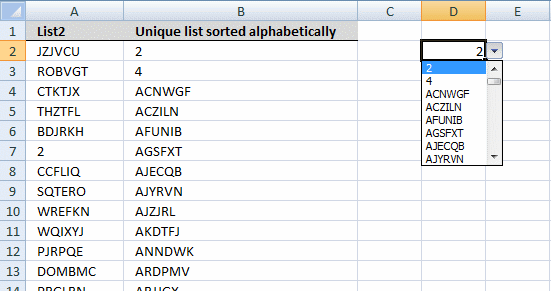Array formula in cell B2:B8000:

=FilterUniqueSort(\$A\$2:\$A\$8212)

How to create array formula

1. Select cell range B2:B8000
2. Type array formula above
3. Press and hold Ctrl + Shift
4. Press Enter once
5. Release all keys

VBA code
You can find the selectionsort function here: Using a Visual Basic Macro to Sort Arrays in Microsoft Excel

```
Function FilterUniqueSort(rng As Range)

Dim ucoll As New Collection, Value As Variant, temp() As Variant
ReDim temp(0)

On Error Resume Next
For Each Value In rng
If Len(Value) &amp;amp;amp;amp;amp;gt; 0 Then ucoll.Add Value, CStr(Value)
Next Value
On Error GoTo 0

For Each Value In ucoll
temp(UBound(temp)) = Value
ReDim Preserve temp(UBound(temp) + 1)
Next Value

ReDim Preserve temp(UBound(temp) - 1)
SelectionSort temp

FilterUniqueSort = Application.Transpose(temp)

End Function

Function SelectionSort(TempArray As Variant)
Dim MaxVal As Variant
Dim MaxIndex As Integer
Dim i, j As Integer

For i = UBound(TempArray) To 0 Step -1

MaxVal = TempArray(i)
MaxIndex = i

For j = 0 To i
If TempArray(j) &amp;amp;amp;amp;amp;gt; MaxVal Then
MaxVal = TempArray(j)
MaxIndex = j
End If
Next j

If MaxIndex &amp;amp;amp;amp;amp;lt; i Then
TempArray(MaxIndex) = TempArray(i)
TempArray(i) = MaxVal
End If
Next i

End Function

```

Where to copy vba code?

1. Press Alt + F11
2. Insert a module into your workbook
3. Copy (Ctrl + c) above code into the code window